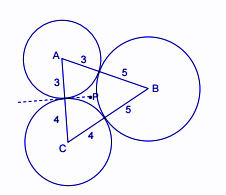QUESTION

# Three circles of radius $3,4,5$ touch each other externally. Tangents are drawn at the point of contact, which meet at $P$. Find the distance between point of contact and $P.$

Hint: Here we will be using the property of radical axis i.e. the axis of two circles is the locus of a point which moves such that the lengths of the tangents drawn from it to the two circles are equal and $r = \dfrac{\Delta }{s} = \dfrac{{\sqrt {s(s - a)(s - b)(s - c)} }}{s}$ . Use this to find the distance between point of contact and $P.$According to question it is given that there are three circles of radius $3,4,5$ and they touch each other externally and if $P$ is the point of intersection of tangents to these circles , we have to find the distance between point of contact and $P.$
Now, Let $A,B$ and $C$ be the centers of the circle respectively.
We know that the line $AP$ bisects the angle formed by the sector at center $A$ .
Similarly we can say this for $BP$ and $CP$.
Clearly, the point $P$ is the in-center of the $\Delta ABC$ and therefore,
$r = \dfrac{\Delta }{s} = \dfrac{{\sqrt {s(s - a)(s - b)(s - c)} }}{s}$$= \sqrt {\dfrac{{\left( {s - a} \right)\left( {s - b} \right)\left( {s - c} \right)}}{s}}$
Now,
$2s = 7 + 8 + 9 = 24$ that is $s = 12$
Thus,
$r = \sqrt {\dfrac{{5 \times 4 \times 3}}{{12}}} = \sqrt {\dfrac{{60}}{{12}}} = \sqrt 5$
Hence, the distance is $\sqrt 5$ units.
Note: Whenever we come up with such questions , we need to apply the basic properties of radical axis i.e. the radical axis and common chord are identical, the radical axis of three circles taken in pairs are concurrent and the radical axis bisects the common tangents. We will use these properties and draw the diagram and then apply the formula.